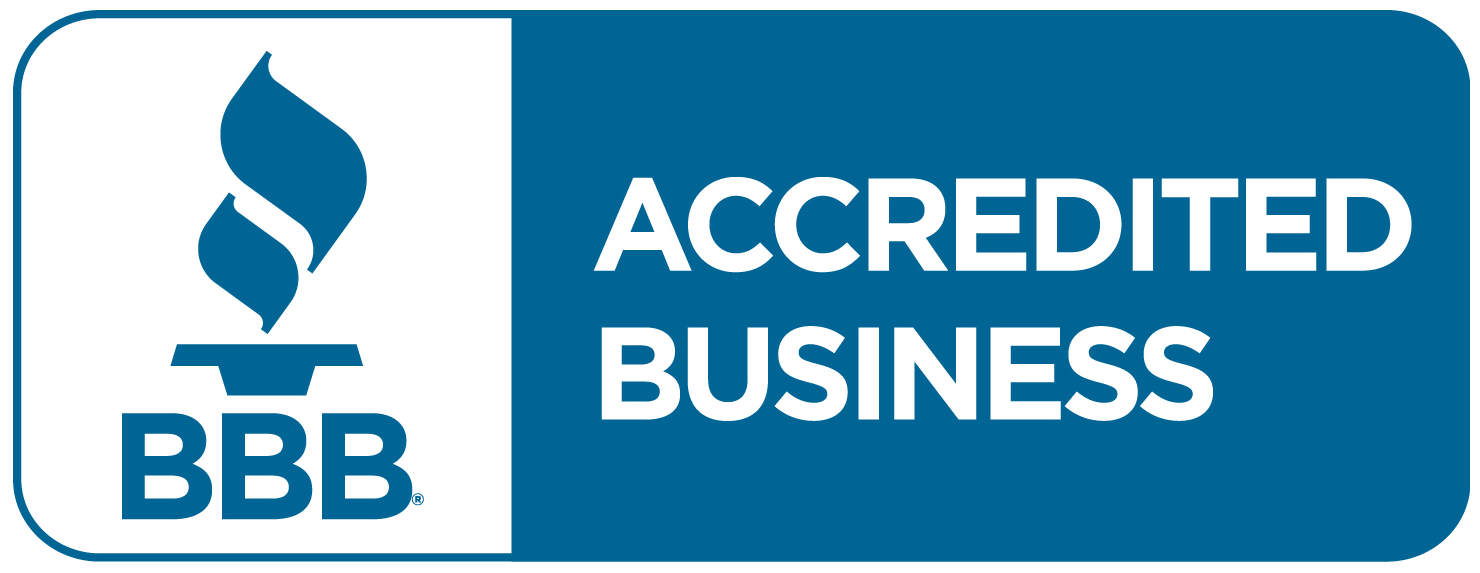Average Cost
Average Cost

## Average Cost Definition

Average cost per unit of production is equal to total cost of production divided by the number of units produced. It is also known as the unit cost. Especially over the long-term, average cost normalizes the cost per unit of production. It also smooths out fluctuations caused by seasonal demand changes or differing levels of production efficiency.

## Average Cost Per Unit Formula

Use the following formula to calculate average cost per unit:

Average Cost Per Unit = Total Production Cost / Number of Units Produced

## Minimization

A company producing goods wants to minimize the average cost of production. The company also wants to determine the cost-minimizing mix and the minimum efficient scale. Companies with a lower average cost per unit of production are better able to defend against aggressive price-cutting among industry competitors than companies with a higher average cost per unit of production.
The cost-minimizing mix is the lowest cost input-output production mix, or the point at which a company can produce the most output for the least cost. This mix occurs at the point of tangency between the isoquant and isocost lines. In economics terminology, the isoquant line is the line that represents all different combinations of production inputs that produce the same quantity of output. In addition, the isocost line represents all possible combinations of production variables that add up to the same level of cost. The point of intersection between the isoquant and isocost lines is the point of cost minimization.
The minimum efficient scale is scale of production at which average cost of production reaches its minimum point. Up to a certain point, more production volume reduces the cost per unit of production. This is economies of scale. The more output that is produced, the more thinly spread the fixed costs of production across the units of output are. This ends up lowering the cost per unit. Furthermore, production economies of scale can lower the threat of new entrants (competitors) into the industry.

## Accounting

In accounting, to find the average cost, divide the sum of variable costs and fixed costs by the quantity of units produced. It is also a method for valuing inventory. In this sense, compute it as cost of goods available for sale divided by the number of units available for sale. This will give you the average per-unit value of the inventory of goods available for sale.ARTICLES YOU MIGHT LIKE

### Financial Ratios

See also: Quick Ratio Analysis Price to Book Value Analysis Price Earnings Growth Ratio Analysis Time Interest Earned Ratio Analysis Use of Financial Ratios Financial Ratios are used to measure financial performance against standards. Analysts compare financial ratios to industry averages (benchmarking), industry standards or rules of thumbs and against internal trends (trends analysis). The

### Margin vs Markup

See Also: Gross Profit Margin Analysis Retail Markup Chart of Accounts (COA) Margin Percentage Calculation Markup Percentage Calculation Margin vs Markup Differences Is there a difference between margin vs markup? Absolutely. More and more in today’s environment, these two terms are being used interchangeably to mean gross margin, but that misunderstanding may be the menace

### Markup Percentage Calculation

See Also: Margin vs Markup Margin Percentage Calculation Retail Markup Gross Profit Margin Ratio Analysis Operating Profit Margin Ratio Analysis Markup Percentage Definition Define the markup percentage as the increase on the cost price. The markup sales are expressed as a percentage increase as to try and ensure that a company can receive the proper

MARCH 28TH-31ST 2022

THE ART OF THE CFO®

Days
Hours
Min

Feb 27th - March 2nd, 2023Days
Hours
Min

JOIN THE NEXT STRATEGIC CFO™ WORKSHOP SERIES

The Art Of The CFO®

Days
Hours
Min

February 27 - March 2, 2023